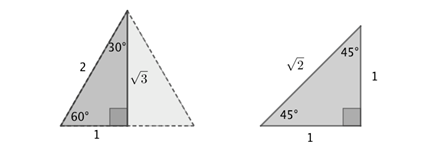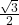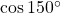# Exact Values of Trig Ratios

An exact value can be:

• an integer;
• a fractionwhereandare integers,;
• a terminating decimal;
• an irrational number given in symbolic form.

With the exception of very few angles such as 0, 90, multiples of 90 – most trig values are irrational. Many trig values can be written as algebraic numbers – that is, a number with a radical. The algebraic form is an exact form and lets us proceed with calculations without losing accuracy. The most common can be calculated from these two triangles, also known as ‘exact value triangles’:The first is simply an equilateral triangle with side length 2, cut in half. The second is an isosceles right angled triangle where the two equal sides measure 1. The other side lengths are calculated using Pythagoras’ Theorem.

We can ‘read’ trig values for angles 60, 30 and 45 from these diagrams using SOH CAH TOA:Now,is an irrational number, however in this form the value is considered exact. We say ‘the exact value ofis. The decimal 0.87 is not considered exactly equal to, even if given to 10 or 100 decimal places.

We can now find exact values for trig values of all multiples of 30 and 45 degrees.

## Example

CalculateCosine is thecoordinate of a point on the circumference of the unit circle.

We notice that 150 degrees belongs in the second quadrant. Cosine is negative in the second quadrant (thecoordinate) and so the valuewill be negative.Next we calculate the reference angle: the acute angle made with the horizontal is 30 degrees.

Therefore the cosine of 150 degrees will be the same but negative of the cosine of 30 degrees. We refer to the exact value triangle above to ‘read’ the cosine of 30 degrees.Try typing bothandon your calculator to ensure that no errors have been made.

# The Unit Circle with Exact Values

The unit circle is often presented with the exact values of cosine and sine for multiples of 30 and 45 – thecoordinates of 16 different points around the circumference. This link takes you to a nice one and comes with a variety of methods for remembering all the values.

# Practice

Use either a printed unit circle or the exact value triangles to practice: